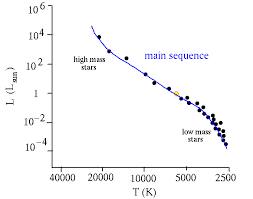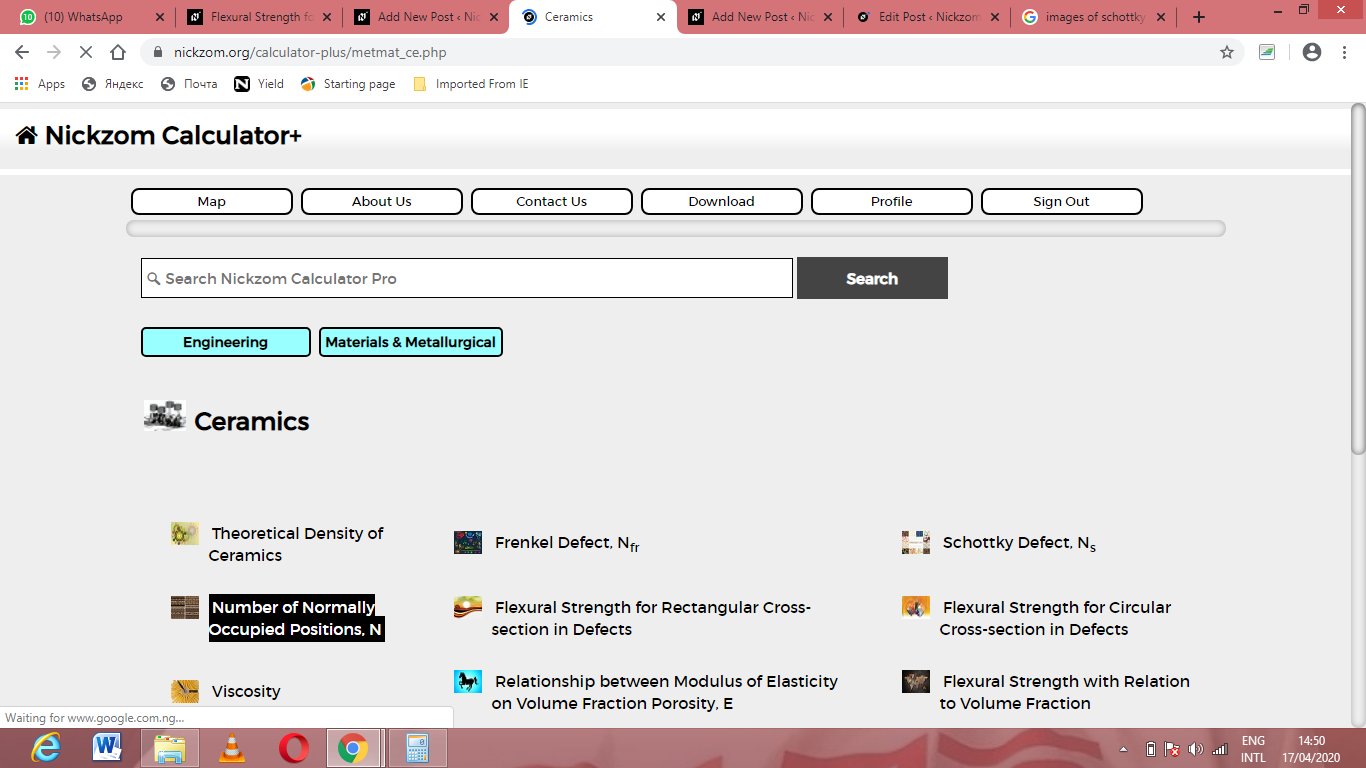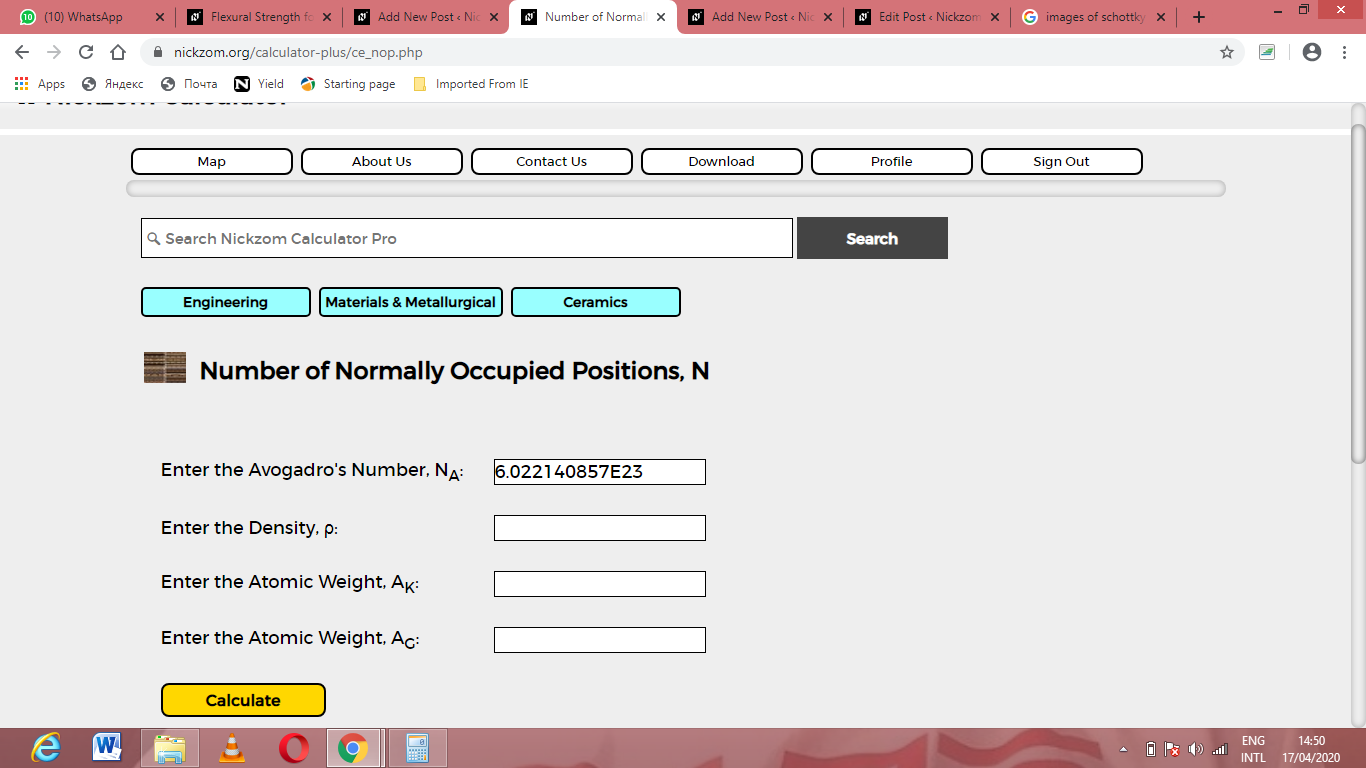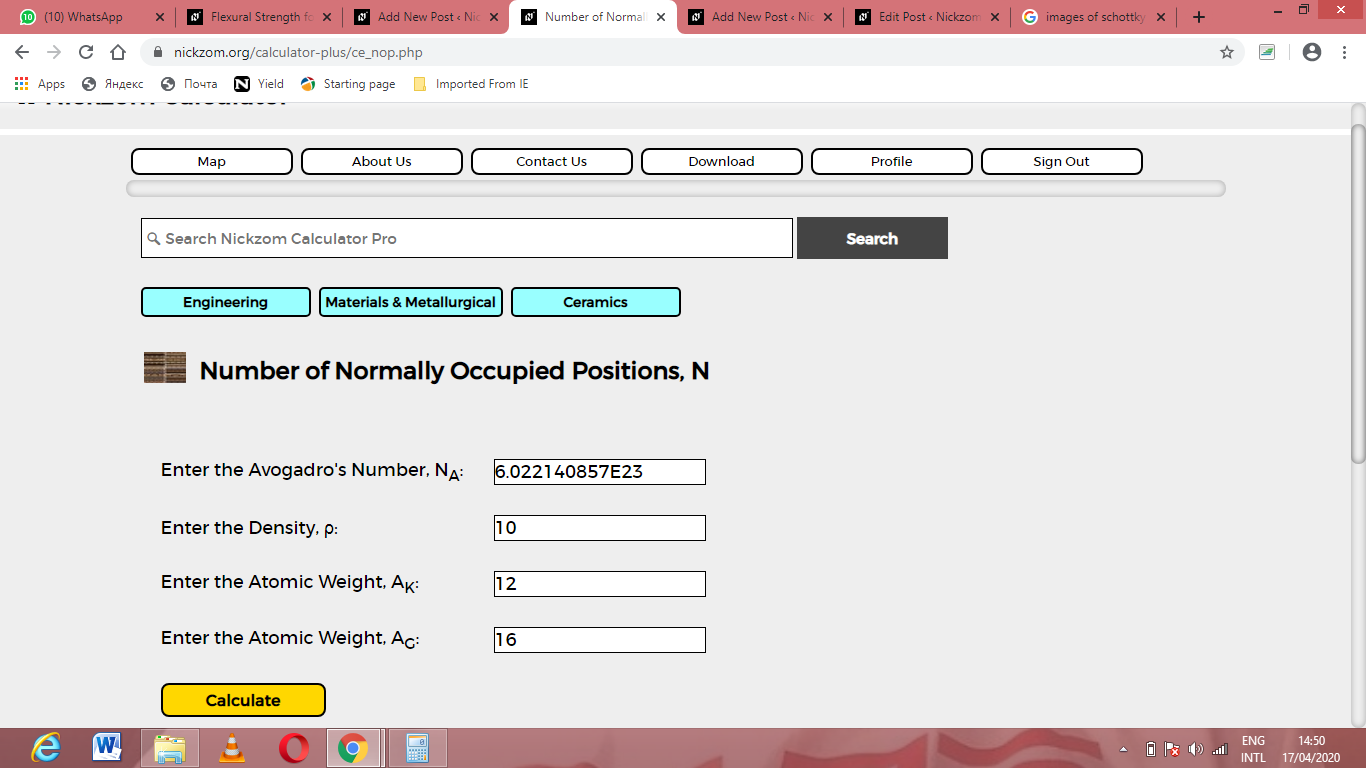# How to Calculate and Solve for Normally Occupied Positions | CeramicsThe image above represents normally occupied positions.

To compute for normally occupied positions, four essential parameters are needed and these parameters are Avogadro’s number (NA), Density (ρ), Atomic weight (AK) and Atomic weights (AG).

The formula for calculating normally occupied positions:

N = NAρ / (AK + AG)

N = Normally Occupied Positions
AK, AG = Atomic Weights
ρ = Density

Let’s solve an example;
Find the normally occupied positions when the avogadro’s number is 6.022e+23, atomic weight is 12, atomic weight is 16 and the density is 10.

This implies that;

NA = Avogadro’s Number = 6.022e+23
AK, AG = Atomic Weights = 12, 16
ρ = Density = 10

N = NAρ / (AK + AG)
N = (6.022e+23)(10)/(12 + 16)
N = (6.022e+24)/(28)
N = 2.15e+23

Therefore, the normally occupied positions is 2.15e+23.

Calculating the Density when the Normally Occupied Positions, the Avogadro’s Number, the Atomic Weights is Given.

ρ = N (AK + AG) / NA

Where;

ρ = Density
N = Normally Occupied Positions
AK, AG = Atomic Weights

Let’s solve an example;
Given that normally occupied positions is 20, the avogadro’s number is 6.022e+23, atomic weights is 18, 6.

This implies that;

N = Normally Occupied Positions =20
NA = Avogadro’s Number = 6.022e+23
AK, AG = Atomic Weights = 18, 6

ρ = N (AK + AG) / NA
ρ = 20 (18 + 6) / 6.022e+23
ρ = 20 (24) / 6.022e+23
ρ = 480 / 6.022e+23
ρ = 7.970e-22

Therefore, the density is 7.970e-22.

Calculating the Atomic Weight (AK) when the Normally Occupied Positions, the Avogadro’s Number, Density and Atomic Weight (AG) is Given.

AK = NAρ / N – AG

Where;

ρ = Density
N = Normally Occupied Positions
AK, AG = Atomic Weights

Let’s solve an example;
Find the atomic weight when the normally occupied positions is 24, the density is 12, the avogadro’s number is 6.022e+23 and the atomic weight is 10.

This implies that;

ρ = Density = 12
N = Normally Occupied Positions = 24
NA = Avogadro’s Number = 6.022e+23
AG = Atomic Weights = 10

AK = NAρ / N – AG
AK = 6.022e+23 x 12 / 24 – 10
AK = 7.2264e+24 / 24 – 10
AK = 3.011e-23 – 10
AK = – 6.98e-23

Therefore, the atomic weight is – 6.98e-23.

Calculating the Atomic Weight (AG) when the Normally Occupied Positions, the Avogadro’s Number, Density and Atomic Weight (AK) is Given.

AG = NAρ / N – AK

Where;

ρ = Density
N = Normally Occupied Positions
AK, AG = Atomic Weights

Let’s solve an example;
Find the atomic weight when the normally occupied positions is 10, the density is 10, the avogadro’s number is 6.022e+23 and the atomic weight is 4.

This implies that;

ρ = Density = 10
N = Normally Occupied Positions = 10
NA = Avogadro’s Number = 6.022e+23
AG = Atomic Weights = 4

AG = NAρ / N – AK
AG = 6.022e+23 x 10 / 10 – 4
AG = 6.022e+24 / 10 – 4
AG = 6.022e-23 – 4
AG = 2.022e-23

Therefore, the atomic weight is 2.022e-23.

Nickzom Calculator – The Calculator Encyclopedia is capable of calculating the normally occupied positions.

To get the answer and workings of the normally occupied positions using the Nickzom Calculator – The Calculator Encyclopedia. First, you need to obtain the app.

You can get this app via any of these means:

To get access to the professional version via web, you need to register and subscribe for NGN 1,500 per annum to have utter access to all functionalities.
You can also try the demo version via https://www.nickzom.org/calculator

Apple (Paid) – https://itunes.apple.com/us/app/nickzom-calculator/id1331162702?mt=8
Once, you have obtained the calculator encyclopedia app, proceed to the Calculator Map, then click on Materials & Metallurgical under Engineering.Now, Click on Ceramics under Material & MetallurgicalNow, Click on Normally Occupied Positions under CeramicsThe screenshot below displays the page or activity to enter your values, to get the answer for the normally occupied positions according to the respective parameters which are the Avogadro’s number (NA), Density (ρ), Atomic weight (AK) and Atomic weights (AG).Now, enter the values appropriately and accordingly for the parameters as required by the Avogadro’s number (NA) is 6.022e+23, Density (ρ) is 10, Atomic weight (AK) is 12 and Atomic weights (AG) is 16.Finally, Click on CalculateAs you can see from the screenshot above, Nickzom Calculator– The Calculator Encyclopedia solves for the normally occupied positions and presents the formula, workings and steps too.